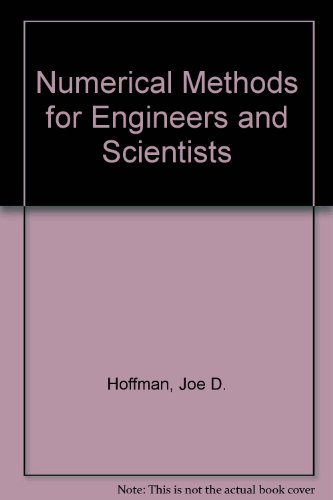## Numerical Methods for Engineers and Scientists: Solutions Manual With Software

### HoffmanView all copies of this ISBN edition:

This text is for courses in numerical methods offered in departments of civil or mechanical engineering and departments of mathematics. Its objective is to introduce the engineer and scientist to numerical methods used to solve mathematical problems that cannot be solved by exact methods. With the general accessibility of highspeed digital computers, it is now possible to obtain rapid and accurate solutions. Mastery of the material presented in this book will prepare engineers and scientists to solve many of their everyday problems, give them insight to recognize when other methods are required, and give them the necessary background to study additional methods. Part I discusses the Basic Tools of Numerical Analysis and considers many of the basic problems that arise in all branches of engineering and science. Part II is devoted to the numerical solution of ordinary differential equations (ODEs). Part III covers the numerical solution of partial differential equations (PDEs). The approach taken is to introduce a type of problem, present sufficient background to understand the problem and possible methods of solution, develop one or more numerical methods for solving the problem, and illustrate the numerical methods with examples. In most cases, the numerical methods presented to solve a particular problem proceed from simple methods to complex methods.

"synopsis" may belong to another edition of this title.

(No Available Copies)

Search Books:

Create a Want

If you know the book but cannot find it on AbeBooks, we can automatically search for it on your behalf as new inventory is added. If it is added to AbeBooks by one of our member booksellers, we will notify you!

Create a Want